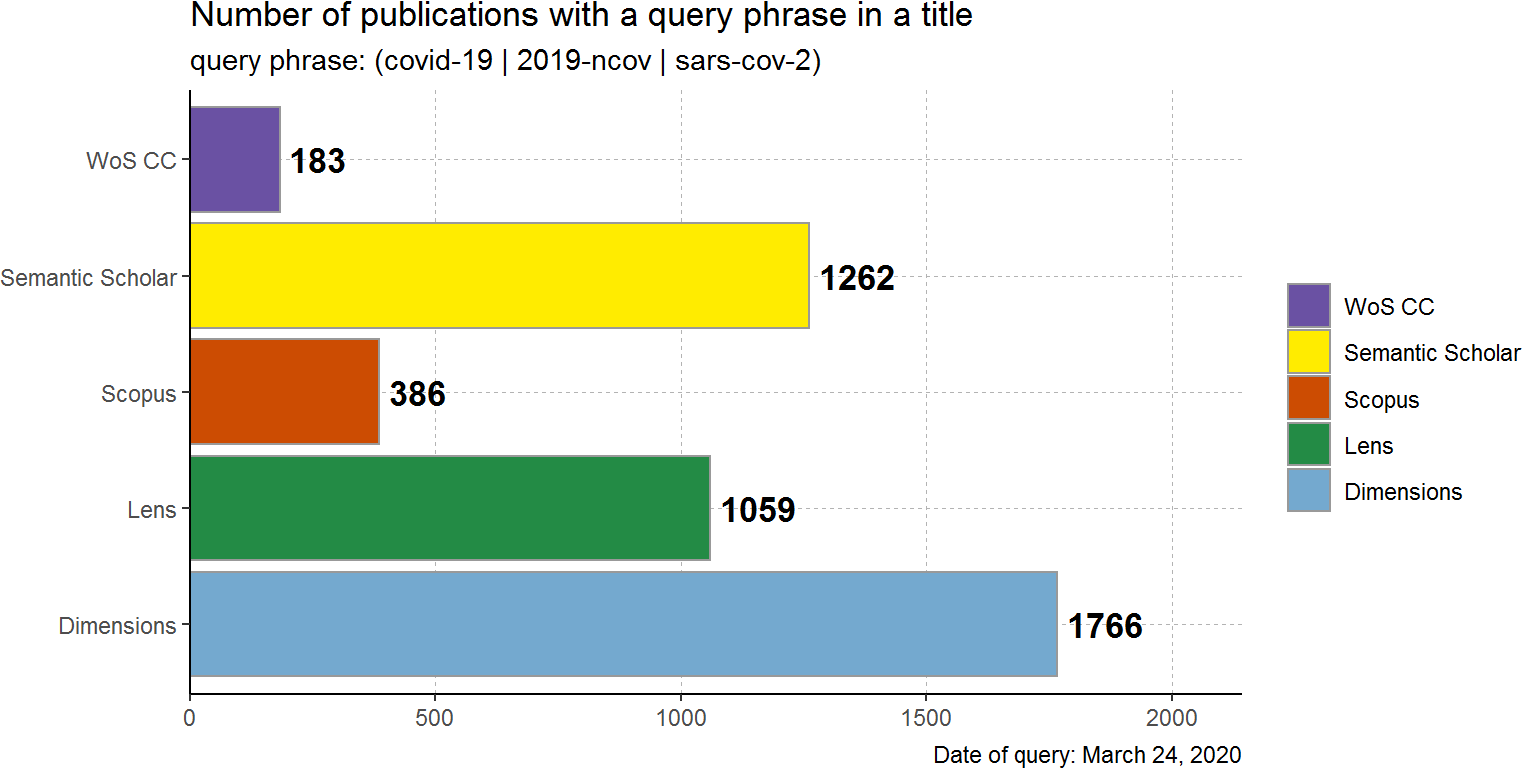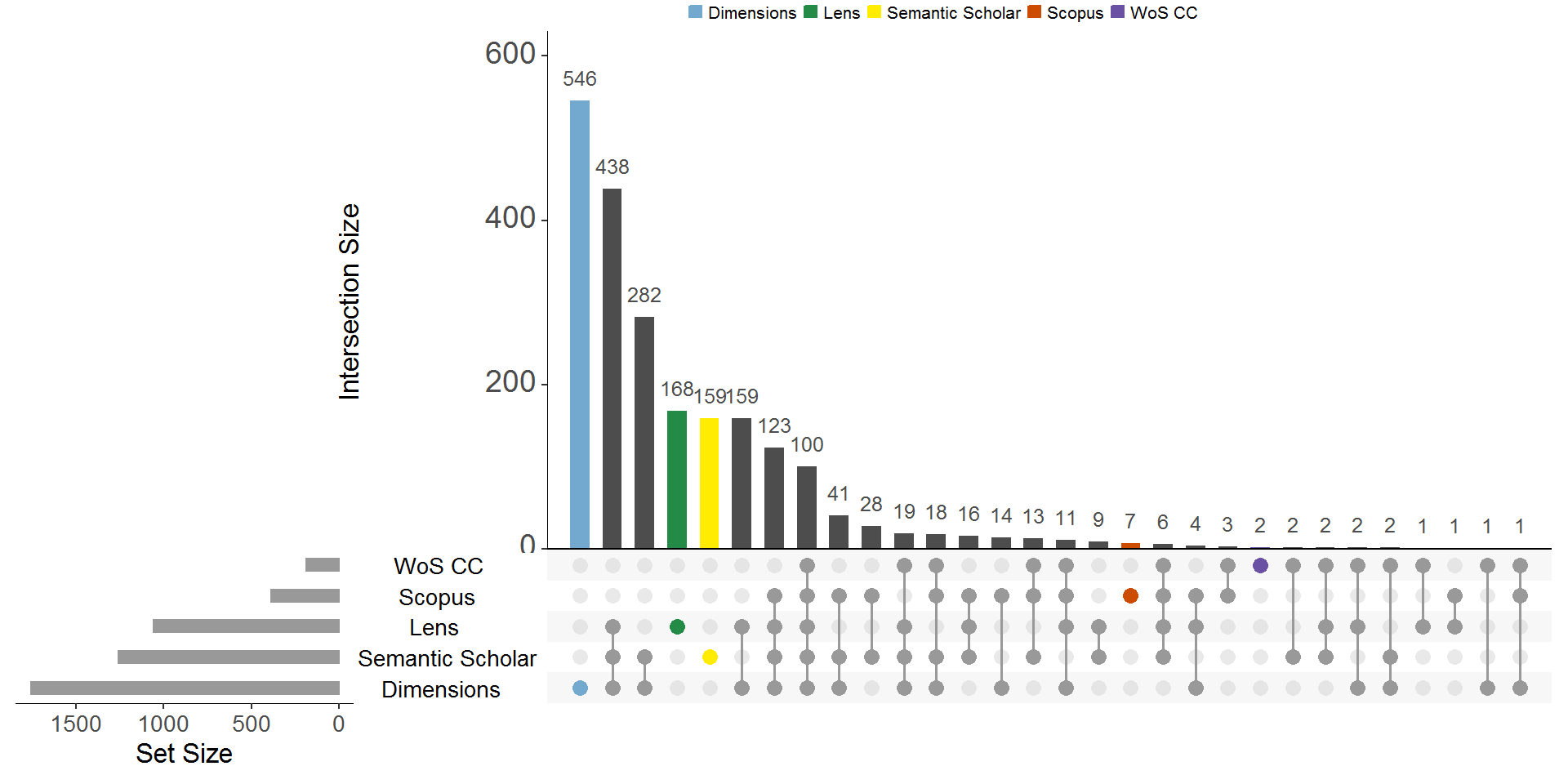COVID-19 raised many questions about availability of scientific knowledge. Even though I am personally sceptic about potential of so-called citizen science to fight such threats as the viruses, the hard times provide us with many examples of why “public” and “open” are so much great comcepts in this world (so far).

## Public datasets

There are few datasets of academic publications that, updated & supported:

I would like to compare a coverage of these datasets to see how many unique publications is offered by each dataset and what are those documents. In order to do that I am going to test these datasets with a plain query.

Query: “covid-19” OR “2019-ncov” OR “sars-cov-2”, in the document title.

## Proprietory search engines

What I am also curious about is whether Web of Science and Scopus (gold standard search engines) are competitive to the public datasets. Do they index the publications fast enough? Is their coverage wide enough? To answer these questions I performed the searches in Scopus and Web of Science and extract the results.

• Scopus: TITLE(“covid-19” OR “2019-ncov” OR “sars-cov-2”) OR ABS(“covid-19” OR “2019-ncov” OR “sars-cov-2”)

• Web of Science: TI=(“covid-19” OR “2019-ncov” OR “sars-cov-2”) OR TS=(“covid-19” OR “2019-ncov” OR “sars-cov-2”); Timespan: All years; Indexes: SCI-EXPANDED, SSCI, A&HCI, CPCI-S, CPCI-SSH, BKCI-S, BKCI-SSH, ESCI.

# reading Dimensions dataset

# reading wos dataset (fast 5K export, Win/Tab)
wos <- read_delim(paste0(dir, "woscc.txt"), delim = "\t")

# reading scopus dataset (csv export)
scopus <- read_csv(paste0(dir, "scopus.csv"))

## Preparing the data

query <- "covid-19|2019-ncov|sars-cov-2"

dim1 <- dim %>%
select(doi = DOI, title = Title, abs = Abstract, year = PubYear, srctitle = Source title) %>%
filter(grepl(query, title, ignore.case = TRUE)) %>%
mutate(doi = str_squish(tolower(doi))) %>%
filter(!is.na(doi)) %>%
mutate(dataset = "Dimensions") %>% unique()

lens1 <- lens %>%
select(doi = DOI, title = Title, abs = Abstract, year = Publication Year, srctitle = Source Title) %>%
filter(grepl(query, title, ignore.case = TRUE)) %>%
mutate(doi = str_squish(tolower(doi)))%>%
filter(!is.na(doi)) %>%
mutate(dataset = "Lens") %>% unique()

ss1 <- ss %>%
select(doi, title, abs = abstract, year = publish_time, srctitle = journal) %>%
filter(grepl(query, title, ignore.case = TRUE)) %>%
mutate(doi = str_squish(tolower(doi)))%>%
mutate(year = substr(year, 1,4)) %>%
filter(!is.na(doi)) %>%
mutate(dataset = "Semantic Scholar") %>% unique()

wos1 <- wos %>%
select(doi = DI, title = TI, abs = AB, year = PY, srctitle = SO) %>%
filter(grepl(query, title, ignore.case = TRUE)) %>%
mutate(doi = str_squish(tolower(doi)))%>%
filter(!is.na(doi)) %>%
mutate(dataset = "WoS CC") %>% unique()

scopus1 <- scopus %>%
select(doi = DOI, title = Title, abs = Abstract, year = Year, srctitle = Source title) %>%
filter(grepl(query, title, ignore.case = TRUE)) %>%
mutate(doi = str_squish(tolower(doi)))%>%
filter(!is.na(doi)) %>%
mutate(dataset = "Scopus") %>% unique()

data <- rbind(dim1, ss1, lens1, wos1, scopus1)

colorset <- c("WoS CC" = "#6a51a3",
"Lens" = "#238b45",
"Scopus" = "#cc4c02",
"Dimensions" = "#74a9cf",
"Semantic Scholar" = "#FFEC00")

I will do just few tests now.

## Number of documents

data %>%
count(dataset) %>%
ggplot(aes(y = dataset, fill = dataset))+
geom_bar(aes(x = n), stat="identity", color = "grey60", size = 0.5) +
geom_text(aes(x = n+20, label = n), hjust = 0, size = 4.5, fontface = "bold")+
labs(title = "Number of publications with a query phrase in a title" ,
x = NULL, y = NULL,
caption = "Date of query: March 24, 2020",
subtitle = paste0("query phrase: (covid-19 | 2019-ncov | sars-cov-2)")) +
scale_fill_manual(name=NULL, values=colorset)+
scale_x_continuous(breaks=pretty_breaks(),
expand = expansion(mult=c(0,0.2)))+
guides(fill = guide_legend(ncol = 1, title.position = "top", reverse = TRUE))+
theme_classic()+
theme(text = element_text(size=11),
panel.grid.major = element_line(size=0.2, linetype = 2, color="grey70"),
panel.grid.minor = element_blank(),
legend.position = "right",
legend.title=element_text(size=rel(0.8)),
legend.text=element_text(size=rel(0.8)),
plot.margin=margin(0,0,0,0))## Intersection by DOI

data %>%
filter(!is.na(doi)) %>%
select(dataset, doi) %>%
mutate(presence=1) %>%
unique() %>%
pivot_wider(id_cols = doi,
names_from = dataset, values_from = presence,
values_fill= list(presence = 0)) %>%
as.data.frame() %>%
upset(sets = c("Dimensions","Lens", "Semantic Scholar", "Scopus", "WoS CC"),
order.by = "freq", decreasing = TRUE, point.size = 3,
query.legend = "top",
text.scale = c(1.5,2,1.5,1.5,1.5,1.5),
main.bar.color = "gray30", sets.bar.color = "gray60", matrix.color = "gray60",
show.numbers = "yes", number.angles = 0, group.by = "degree",
queries = list(
list(query = intersects, params = list("Dimensions"),
color = "#74a9cf", active = T, query.name = "Dimensions"),
list(query = intersects, params = list("Lens"),
color = "#238b45", active = T, query.name = "Lens"),
list(query = intersects, params = list("Semantic Scholar"),
color = "#FFEC00", active = T, query.name = "Semantic Scholar"),
list(query = intersects, params = list("Scopus"),
color = "#cc4c02", active = T, query.name = "Scopus"),
list(query = intersects, params = list("WoS CC"),
color = "#6a51a3", active = T, query.name = "WoS CC")))Not so many unique articles in WoS CC (2) or Scopus (7), whereas 546 in Dimensions, and 168 in Lens, 159 in Kaggle dataset (Semantic Scholar).

And the main question remains - what are those publications present in Dimensions/Lens/SemanticScholar that I failed to retrieve from WoS CC and Scopus. Are they from reliable sources? Can it be “fake science”?

## “Unique” titles

I filtered the DOIs that present in Scopus & WoS CC datasets from a combined Lens/SemantiScholar/Dimensions dataset and counted the journal titles (not the very accurate thing to do with the datasets having empty cells).

wos_scopus_dois <- data %>%
filter(dataset %in% c("Scopus", "WoS CC")) %>%
select(doi) %>% unique()

data %>% filter(!doi %in% wos_scopus_dois\$doi) %>%
select(doi, source_title = srctitle) %>% na.omit() %>%
mutate(source_title = str_squish(tolower(source_title))) %>%
count(source_title, name = "n_of_dois") %>%
arrange(desc(n_of_dois)) %>%
DT::datatable(rownames = FALSE, escape = FALSE,
filter = "none",
options = list(dom = "tp",
columnDefs = list(
list(width = '600px', targets = c(0),
width = '50px',  targets =c(1)))))

Many unique publications emerge from the preprint repositories - medRxiv, bioRxiv, ChemRxiv, SSRN, etc, but there are also some prominent journals here - The Lancet, BMJ, J. of Medical Virology, Radiology, JAMA, NEJM….

Apparently, WoS CC and Scopus are just too slow. Of course, due to their strict indexing rules & policies that bring so much comfort when time doesn’t matter.

## Disclaimer

This post was just an exercise. I was curious about the topic, and decided to share the results. Anyone can download the datasets and continue this study.